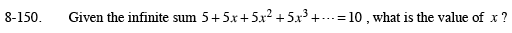### Home > PC > Chapter 8 > Lesson 8.2.6 > Problem8-150

8-150.Use the infinite geometric series formula(shown below) and set it equal to 10. Then solve for x.

$S_{n}=\frac{a_{1}}{1-x}$

a1 = 5

5 = 10(1 − x)
5 = 10 − 10x
−5 = −10x